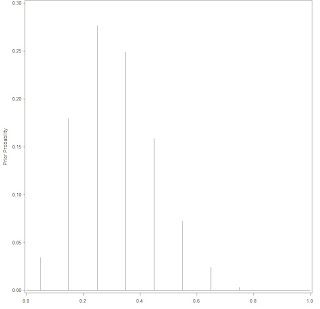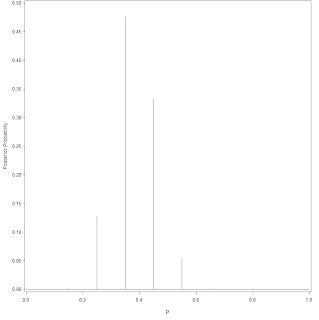## Sunday, February 13, 2011

### Bayesian compution with R examples in SAS

``p = seq(0.05, 0.95, by = 0.1)prior = c(1, 5.2, 8, 7.2, 4.6, 2.1, 0.7, 0.1, 0, 0)prior = prior/sum(prior)data = c(11, 16)post = pdisc(p, prior, data)round(cbind(p, prior, post),2)library(lattice)PRIOR=data.frame("prior",p,prior)POST=data.frame("posterior",p,post)names(PRIOR)=c("Type","P","Probability")names(POST)=c("Type","P","Probability")data=rbind(PRIOR,POST)xyplot(Probability~P|Type,data=data,layout=c(1,2), type="h",lwd=3,col="black")``

``options fullstimer formchar='|----|+|---+=|-/\<>*'  formdlim='-'  error=3;/***************************************************//*模仿oloolo大牛的程序。把 Bayesian compution with R 中的例子用SAS写出来；原文为oloolo所写；用来练习，写不出来的，就看看oloolo怎么写的；哈哈 :)*//*The following is to draw the graph for the discrete prior;There are ten points that p may take, each with its weight respectively;*/data p;    do p = .05 to .95 by .1;        output;    end;run;data prior;input prior @@;cards;1  5.2  8  7.2  4.6  2.1  .7  .1  0  0;run;data p;    retain sum_prior 0;    do until (eof1);        set prior end = eof1;        sum_prior + prior;    end;    do until (eof2);        merge p prior end = eof2;        prior = prior/sum_prior;        output;    end;    drop sum_prior;run;goptions reset = all;symbol interpol=Needle value=none line=1 color=black;axis1 label = (angle = 90 'Prior Probability') order = (0 to 0.3 by .05) minor = none;axis2 label = ('p') order = (0 to 1 by .2) minor = none;proc gplot data = p;    plot prior*p / vaxis=axis1 haxis=axis2;run;quit;````/*Next is to calculate the posterior and then draw it;*/%let n0=16;%let n1=11;/*Calculate the log-likelihood*/data p;   set p; if p > 0 and p < 1 then do;     log_like = log(p)*&n1 + log(1-p)*&n0; end; else     log_like = -999*(p=0)*(&n1 > 0) + (p=1)*(&n0 > 0) ;run;/*Calculate the max(log-likelihood). Since exp(log_likelihood) is very colse to 0, if use it directly, almost allcalculated items is zero. So, use exp(log_likelihood - max), sacle the likelihood. It doesn't change the valueof likelihood since final likelihood = likelihood / sum(likelihood). If both numerator and denominator bothscaled by the same number, it doesn't change the fraction value.*/proc means data = p noprint;    var log_like;    output out = _max  max(log_like)=max;run;data p;    set _max;    sum_like = 0;    do until (eof);        set p end = eof nobs = ntotal;        likelihood = exp(log_like - max);        sum_like + likelihood;    end;    do until (eof1);        set p end = eof1;        likelihood = exp(log_like - max)/sum_like;        posterior = likelihood * prior;        output;    end;run;data p;    sum_post = 0;    do until (eof);        set p end = eof;        sum_post + posterior;    end;    do until (eof2);        set p end =  eof2;        posterior = posterior / sum_post;        output;    end;run;goptions reset = all;symbol interpol=Needle value=none line=1 color=black;axis1 label = (angle = 90 'Posterior Probability') order = (0 to 0.5 by .05) minor = none;axis2 label = ('p') order = (0 to 1 by .2) minor = none;proc gplot data = p;plot posterior*p / vaxis=axis1 haxis=axis2;run;quit;``## Thursday, February 10, 2011

### data set match merge, an example to study zz

The original post want to match merge A and B, and then got the result as below:

X   Y         Z               flag
1   red      brown       0
1   red      red            1
1   blue     brown       0
1   blue     red            0
3   yellow  red            0
3   yellow  pink           0
3   green   red            0
3   green   pink           0

In proc sql, it's not difficult to use inner join to merge A and B conditional on a.x = b.x. And we need to assign value to flag as if a.y matched by b.z.  At last the program can be down as:

``````

data a;
input x y \$;
cards;
1 red
1 blue
3 yellow
3 green
;
run;

data b;
input x z \$;
cards;
1 brown
1 red
2 yellow
3 red
3 pink
;
run;

proc sql;
select a.x, a.y, b.z, case when a.y = b.z then 1 else 0 end as flag
from a, b
where a.x=b.x;
quit;

``````

***************************************************************************
Somebody gives the solution with data step. It's pretty good, as following:

``````

data c;
set a;
do i = 1 to num;
set b(rename = (x = x2)) nobs = num point = i;
output;
end;
run;

data want (drop = x2);
set c;
if y = z then flag = 1;
else flag = 0;
where x = x2;
run;

``````

To make it clearer, here, the print of data set c is:

Obs    x    y         x2    z

1    1    red        1    brown
2    1    red        1    red
3    1    red        2    yellow
4    1    red        3    red
5    1    red        3    pink
6    1    blue       1    brown
7    1    blue       1    red
8    1    blue       2    yellow
9    1    blue       3    red
10    1    blue       3    pink
11    3    yellow     1    brown
12    3    yellow     1    red
13    3    yellow     2    yellow
14    3    yellow     3    red
15    3    yellow     3    pink
16    3    green      1    brown
17    3    green      1    red
18    3    green      2    yellow
19    3    green      3    red
20    3    green      3    pink

## Wednesday, February 9, 2011

### How to draw Normal density in SAS

/* Here we will use function PDF('NORMAL', x, mu, sigma) to get pdf
http://support.sas.com/documentation/cdl/en/lrdict/64316/HTML/default/viewer.htm#a000270634.htm
*/

%let a1=1;
%let b1=1;
%let a2=-1;
%let b2=1;
%let a3=0;
%let b3=1;

data pdf;
do i=-5 to 5 by 0.02;
pdf1=pdf('Normal', i, &a1, &b1);
pdf2=pdf('Normal', i, &a2, &b2);
pdf3=pdf('Normal', i, &a3, &b3);
output;
end;
run;

proc print data=pdf;
run;

goptions reset=all;
symbol1 interpol=j  color=red  width=1  value=none;
symbol2 interpol=j  color=blue width=2  value=none;
symbol3 interpol=j  color=gree width=3  value=none;
axis1   label=(angle=90  'Density'order=(0 to .5 by .1) minor=none;
axis2   label=('i')      order=(-5 to 5 by 1minor=none;;
legend label=none   position=(top right insidemode=share;
proc gplot data=pdf;
plot   pdf1*i  pdf2*i  pdf3*i /overlay
vaxis=axis1
haxis=axis2
legend=legend;